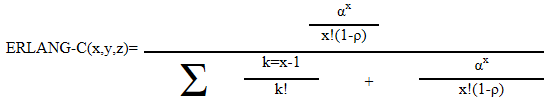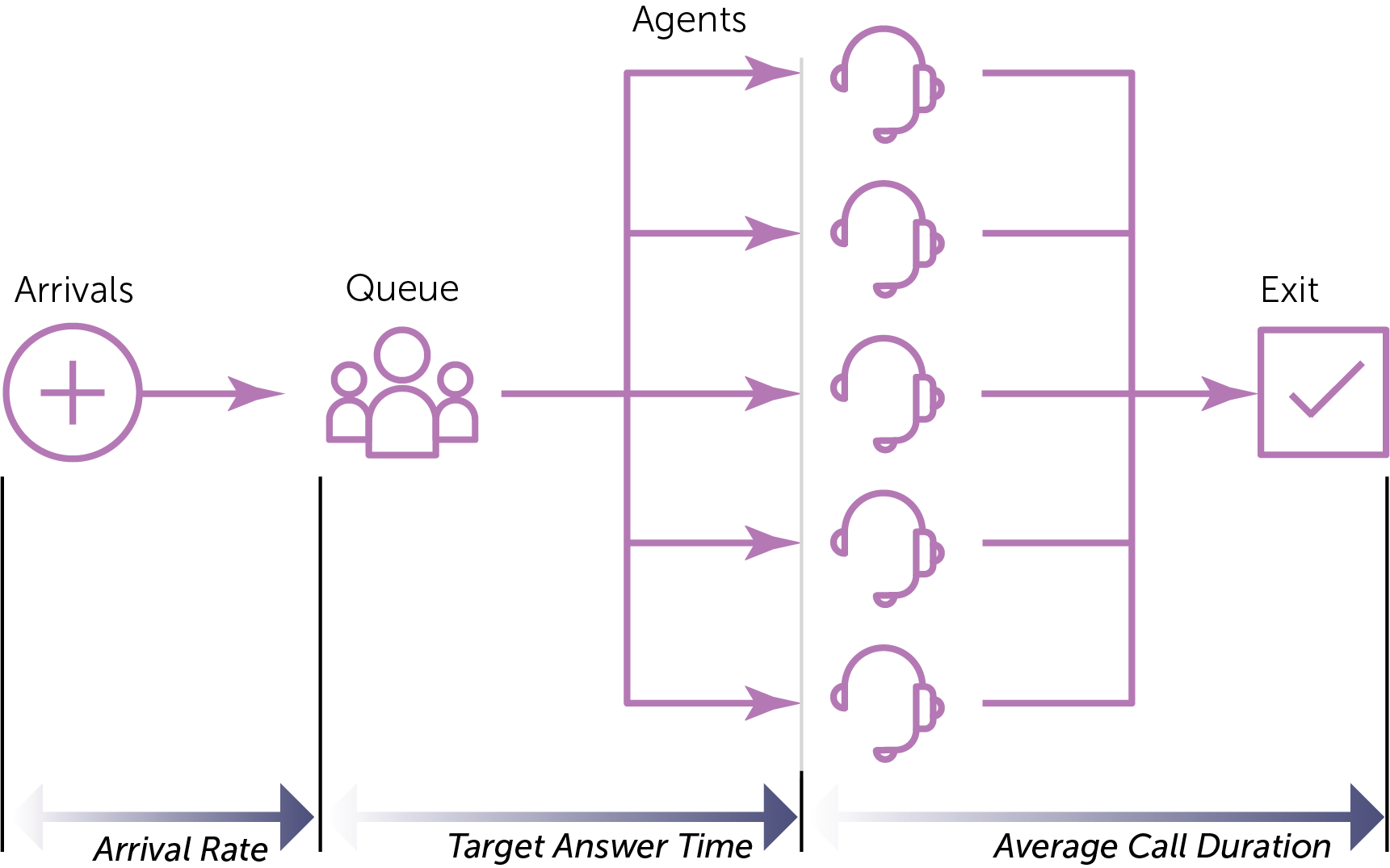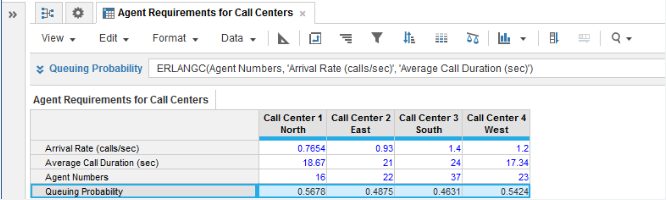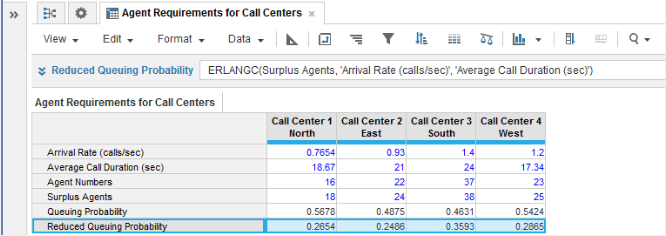1. Calculation functions
2. All Functions
3. Call Center Planning Functions
4. ERLANG-CCalculates the probability a call will be placed in an unlimited queue.

## Syntax

ERLANGC(x, y, z)

where:

• x: number of servers, telephone lines, or agents
• y: arrival rate of calls or the number of calls per unit of time
• z: average call duration

Equation:Where:

• call arrival rate multiplied by the average call duration = y*z
• ρ is intensity, or offered load per agent or server;
• offered load divided by the number of agents = α/x

Flow Diagram:## Format

Input Format Output Format

x: Number

y: Number (time)

z: Number (time)

Number

## Arguments

The function uses the following arguments:

• x: Numeric line item, property, or expression
• y: Numeric line item, property, or expression - Must be the same time unit as used for argument z
• z: Numeric line item, property, or expression - Must be the same time unit as used for argument y

## Constraints

The function has the following constraints:

• All parameters are mandatory.
• All parameters must be a numeric line item, property, or expression.
• Time unit used for the y and z parameters must be the same.
• Result line item must be numeric.
• Maximum number of agents is five million.

## Excel equivalent

• No Excel equivalent

## Example

In this example, we have four call centers each covering a separate geographical area:

• Each call center is modeled under the assumption of automatic queuing of an incoming call, if all agents are occupied.
• Agent staffing levels for each call center are known.
• The arrival rate of incoming calls and the average call duration are known for each call center from historical monitoring of operational performance.

With the baseline metrics entered for each call center in numeric source line items for Agent Numbers, Arrival Rate (calls/sec), and Average Call Duration (sec), we can use ERLANG-C in a Queuing Probability numeric result line item to calculate the probability a call will be placed in a queue:

ERLANGC(Agent Numbers, 'Arrival Rate (calls/sec)', 'Average Call Duration(sec)')If we want to improve on the probability that incoming calls will be placed in a queue—perhaps to bring it down closer to a 0.25 probability—then we can add an alternative numeric source line item for Surplus Agents and then introduce a second ERLANG-C calculation for a Reduced Queuing Probability numeric result line item, allowing us to perform what-if analysis by entering a number of extra agents until we reach the desired queuing probability:

ERLANGC(Surplus Agents, 'Arrival Rate (calls/sec)', 'Average Call Duration(sec)')